# Performance Comparison: JavaScript vs. Python for Machine LearningIn my previous article, I discussed the pros and cons of using JavaScript for machine learning. I delved into whether it performs as well as Python-based solutions on ML tasks. And now, I’ve put the programming language to the test.

I used several models to measure Javascript’s performance in machine learning, benchmarking the actual results against Python-based solutions.

For context: the task we used for the tests was ‘fraudulent financial transactions detection.’

## The Dataset

I chose to use synthetic datasets generated by the PaySim mobile money as they include 6,362,620 records of financial transactions — the datasets comprise eleven columns, and below is a snippet of the data.

 step type amount nameOrigText oldbalanceOrg newbalanceOrig nameDest oldbalanceDest newbalanceDest isFraud isFlaggedFraud 1 PAYMENT 9839.64 C1231006815 170136.0 160296.36 M1979787155 0.0 0.0 0 0 2 PAYMENT 1864.28 C1666544295 21249.0 19384.72 M2044282225 0.0 0.0 0 0 3 TRANSFER 181.00 C1305486145 181.0 0.00 C553264065 0.0 0.0 1 0 4 CASH_OUT 181.00 C840083671 181.0 0.00 C38997010 21182.0 0.0 1 0

The dataset includes:

• 6,354,407 legitimate transactions; and,
• 8,213 fraudulent transactions.

This translates into a 0.1% fraud scale. It’s worth mentioning that fraud only occurs for TRANSFER and CASH_OUT transactions — below, you can find the exact number of transactions per transaction type.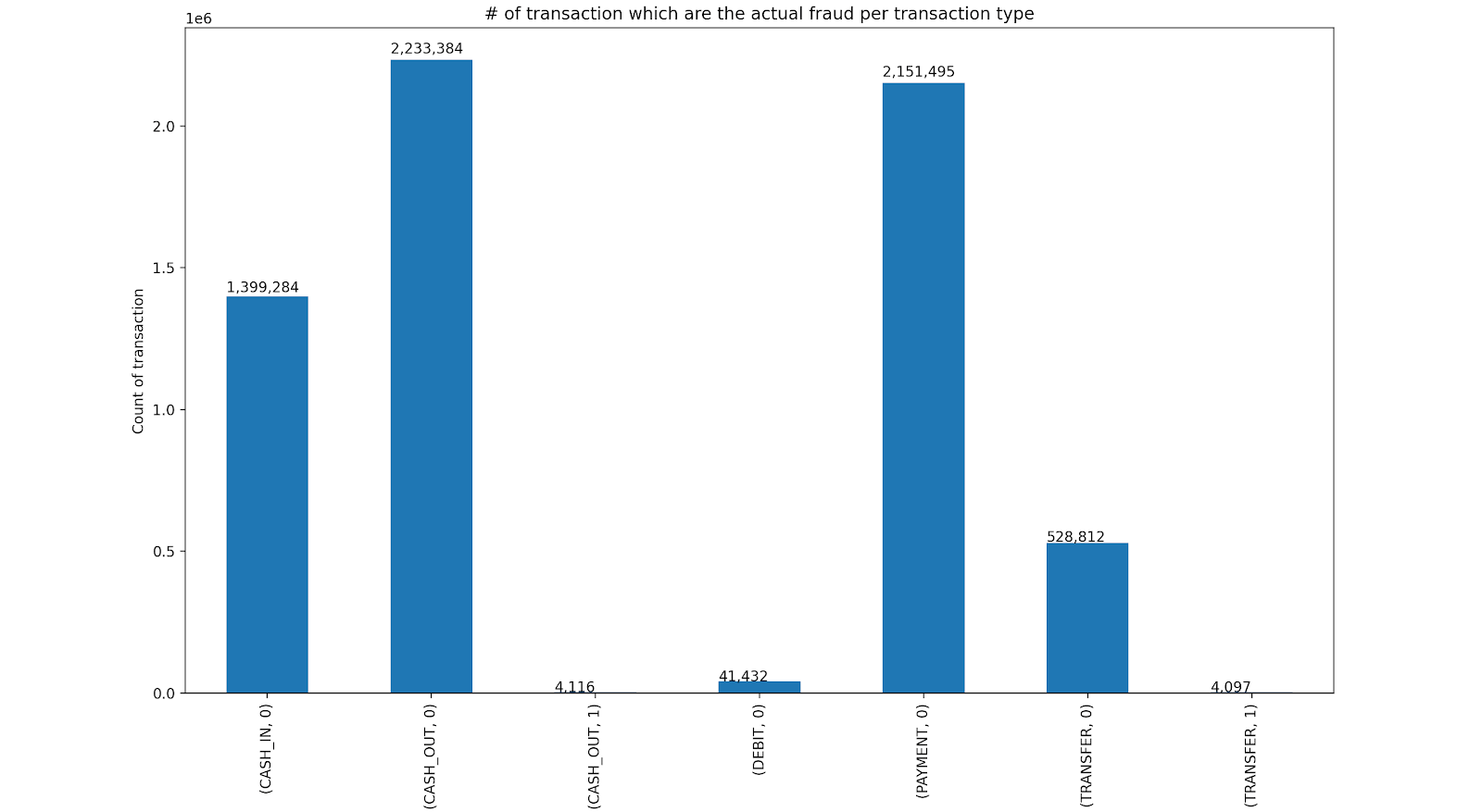We carried out data analysis and following this, deemed, isFlaggedFraud, nameOrig, and nameDest columns as irrelevant to the result — below, you can find a correlation heatmap between the relevant columns.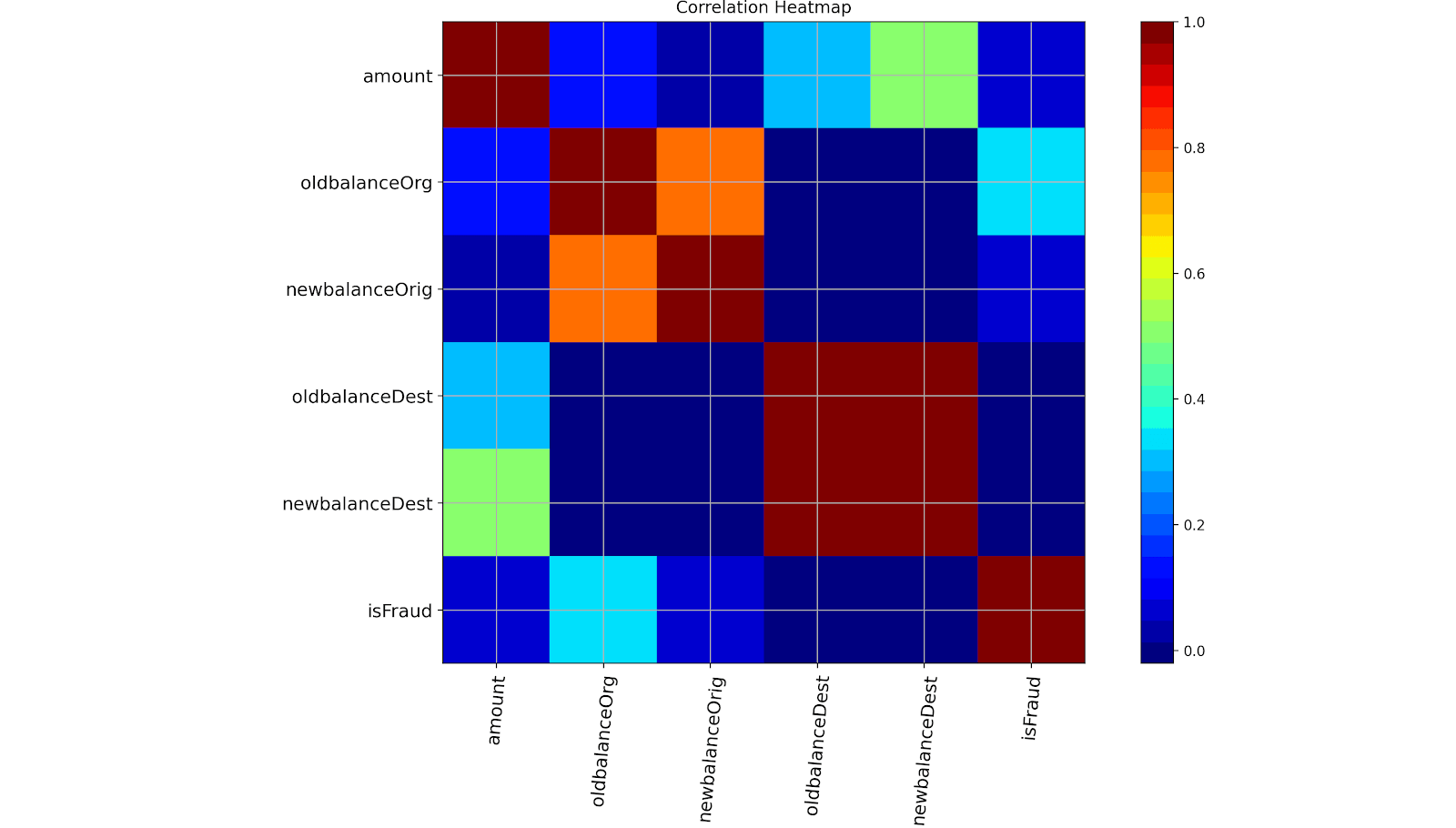## Benchmark Environment And Method

The following gives details about the environment and methods used to benchmark the data.

### Environment

We performed all tests on machines with the following specifications:

• CPU: Intel Core i7-4770HQ, clocked 2.2 GHz
• RAM: 16GB
• GPU: None
• OS: macOS Catalina (10.15.2)

We used the following software environments:

• Node 12.16.1
• Python 3.7.6

We used the following libraries:

• Python: Pandas, NumPy, scikit-learn, Keras
• JavaScript: Zebras, machinelearn.js, fscore, Tensorflow.js, ModelScript

We carried out measurements for the JavaScript code by calculating the time difference between the Date.now() value at the beginning of a function and the end. We used a broadly similar approach for Python with one exception: we used the time function from the time package.

We measure the execution for the following sections in both programs:

• Data read from the file
• Data preprocessing
• Split into test and train sets
• Learning
• Prediction

We chose just three models for the test:

1. Linear Regression
2. Random Forest Classifier
3. Neural Network.

We measured each metric ten times (on datasets that included all records and reduced to 1m records), and the average results are shown below under the heading, ‘Results.’

Before we look at the results, one aspect worth noting when I reproduced the Python code in JavaScript was the libraries’ immaturity. There have been significant improvements over the years here. Nonetheless, I had to spend a lot of time searching through the libraries to get the same functionality I had with the Python version.

In Python, it’s a breeze. It’s a well-recognized language for machine learning. Therefore, the level of community engagement with the development of libraries is many times higher.

## The Results

There are no two ways about it, so let’s cut straight to the chase.

Python wiped the floor with JavaScript — you can see this in the graphs and the full results below.

## The Full Dataset

### Data read from file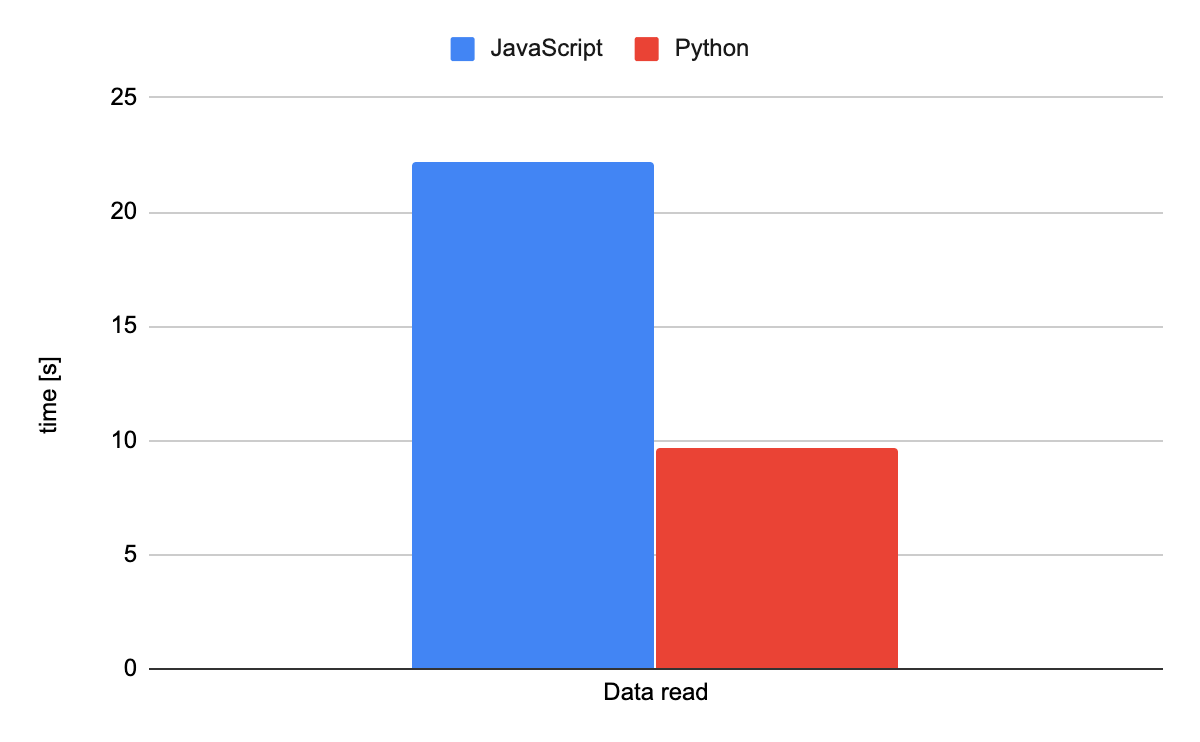Precise results: JavaScript = 22.197 seconds — Python = 9.669 seconds

### Data preprocessing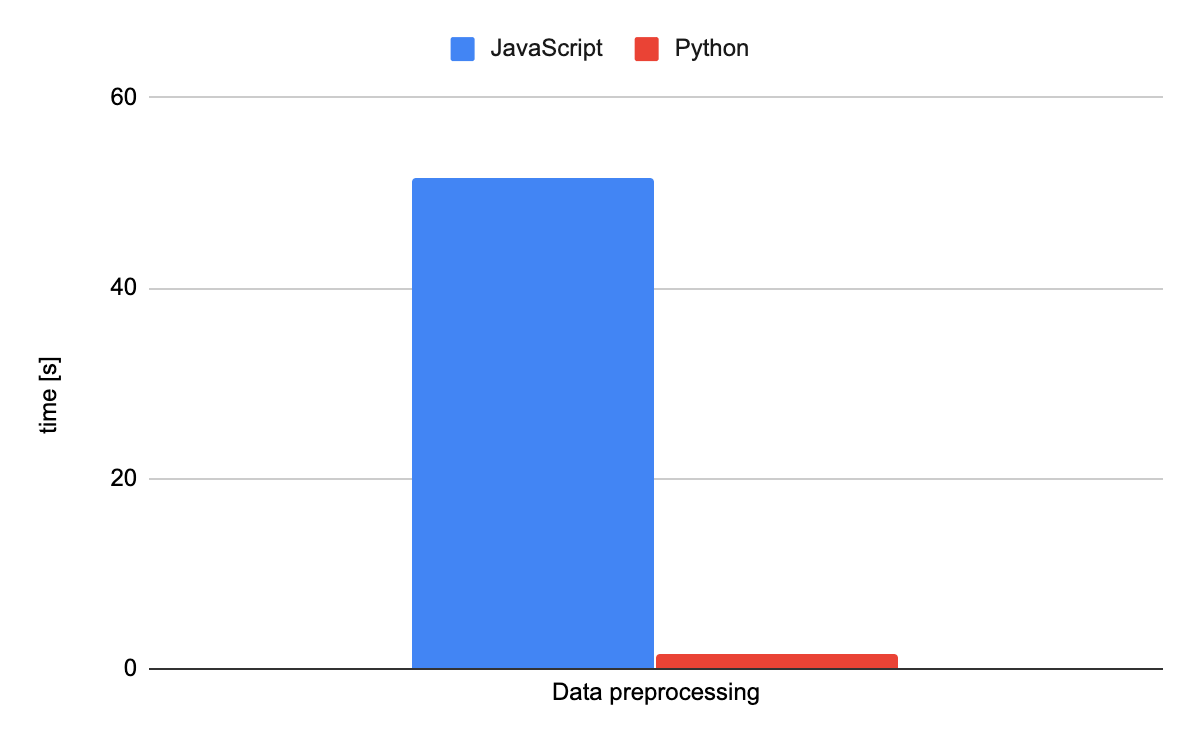Precise results: JavaScript = 51.667 seconds — Python = 1.580 seconds

### Split into test and train sets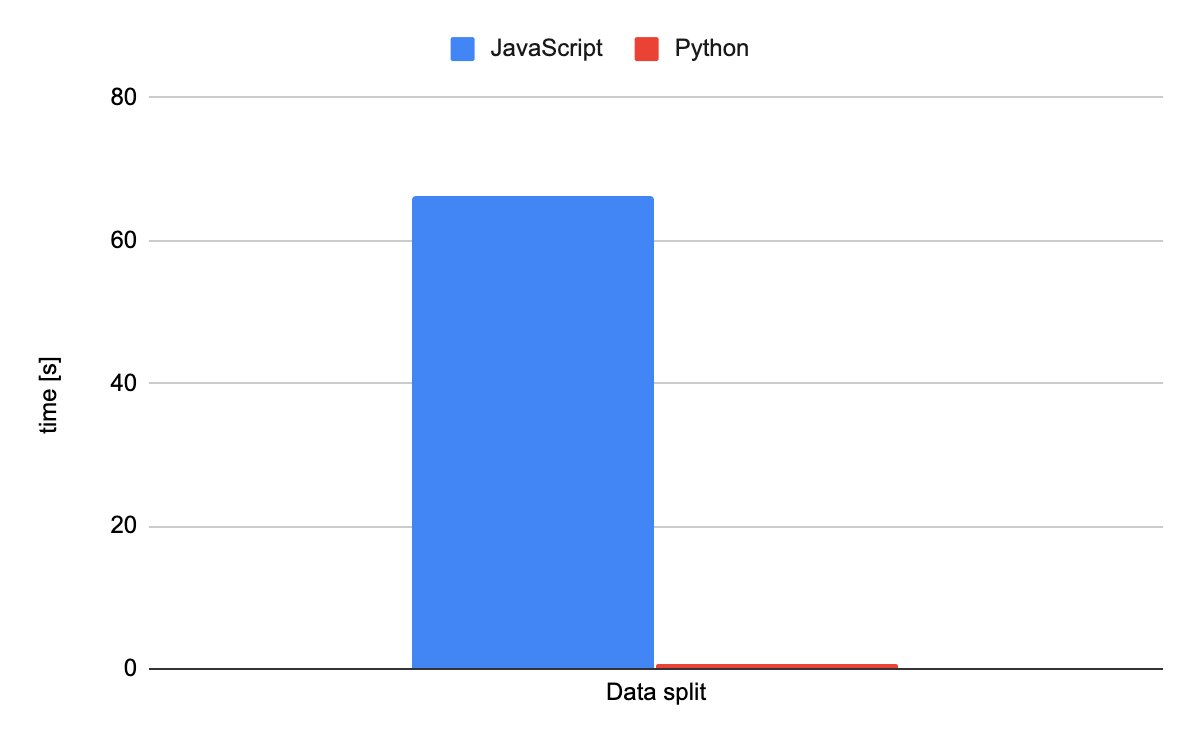Precise results: JavaScript = 66.238 seconds — Python = 0.646 seconds

### Learning and predicting

#### Linear RegressionPrecise results — Training: JavaScript = 193.436 seconds — Python = 4.728 seconds
Precise results — Prediction: JavaScript = 21.535 seconds — Python = 0.034 seconds

### Random forest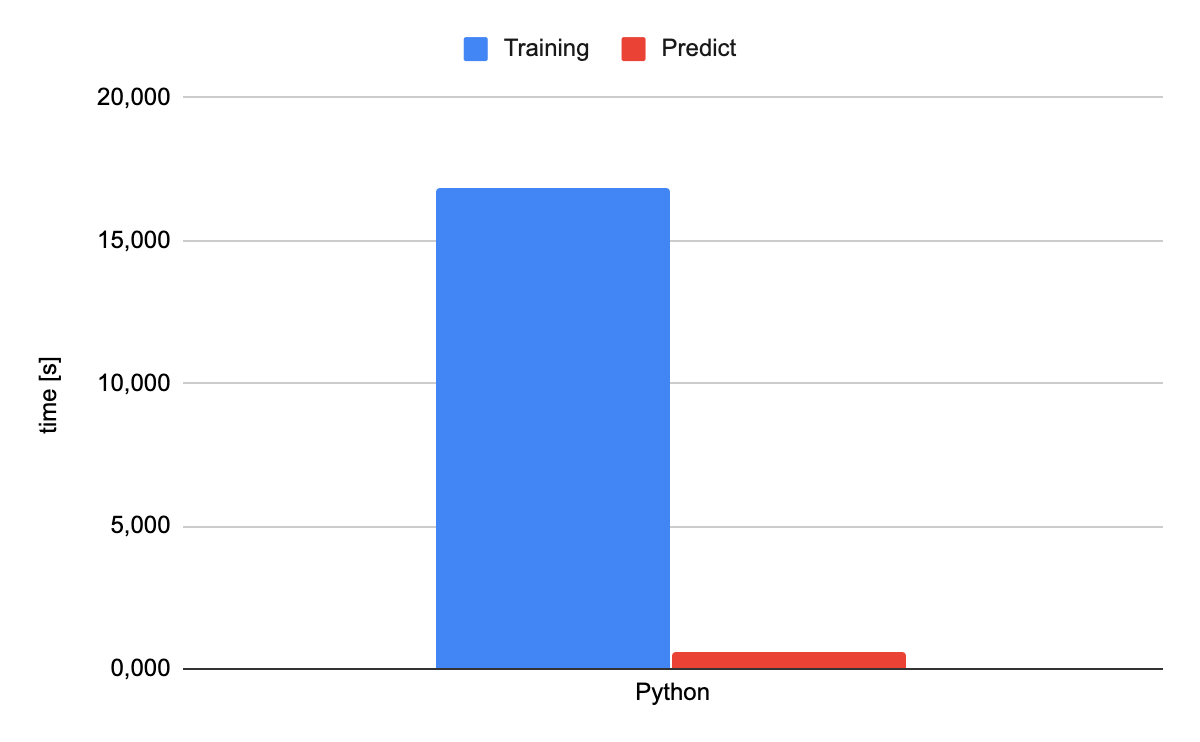Precise results: Python Training = 16.854 seconds — Python Prediction = 0.588 seconds

No, we didn’t make a mistake. We only got Python results as the truth is… JavaScript never made it.

The process didn’t finish working in the given timeframe.

### Neural network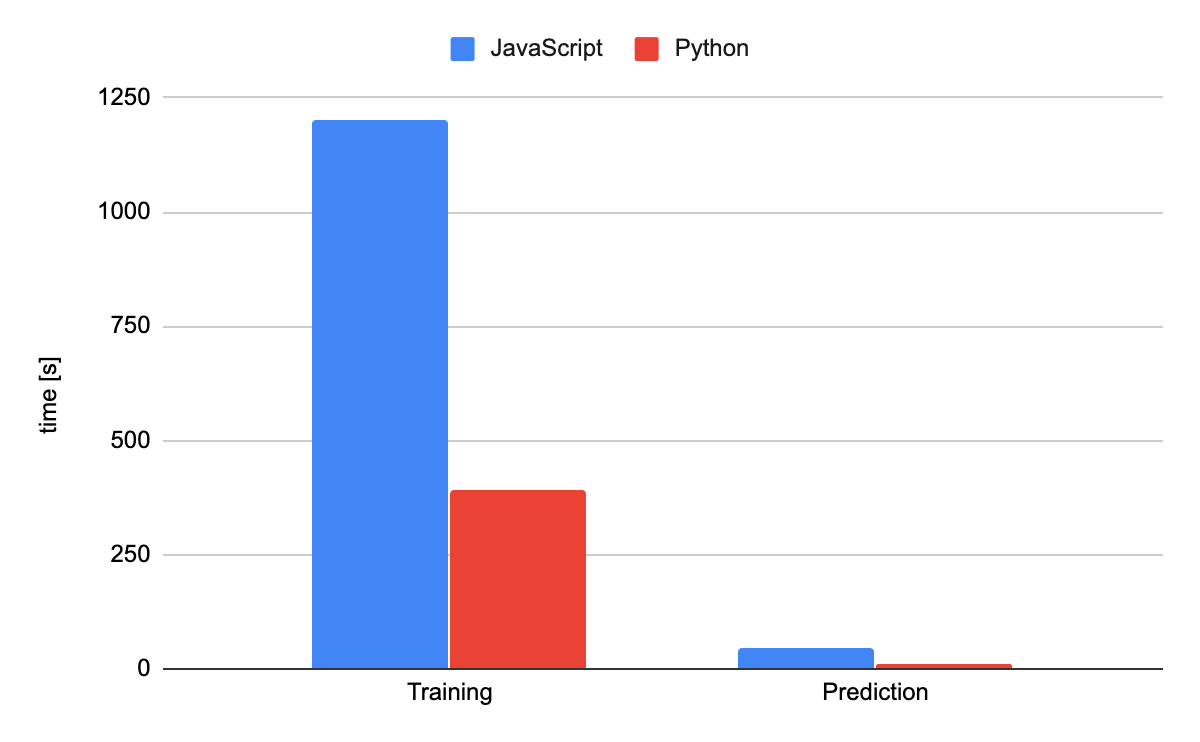Precise results — Training: JavaScript = 1199.665 seconds — Python = 391.072 seconds
Precise results — Prediction: JavaScript = 46.707 seconds — Python = 12.751 secondsPrecise results: JavaScript = 2.148 seconds — Python = 1.537 seconds

### Data preprocessingPrecise results: JavaScript = 6.334 seconds — Python = 0.248 seconds

### Split into test and train setsPrecise results: JavaScript = 7.116 seconds — Python = 0.068 seconds

### Learning and predicting

#### Linear RegressionPrecise results — Training: JavaScript = 30.317 seconds — Python = 0.555 seconds
Precise results — Prediction: JavaScript = 1.942 seconds — Python = 0.004 seconds

### Random forest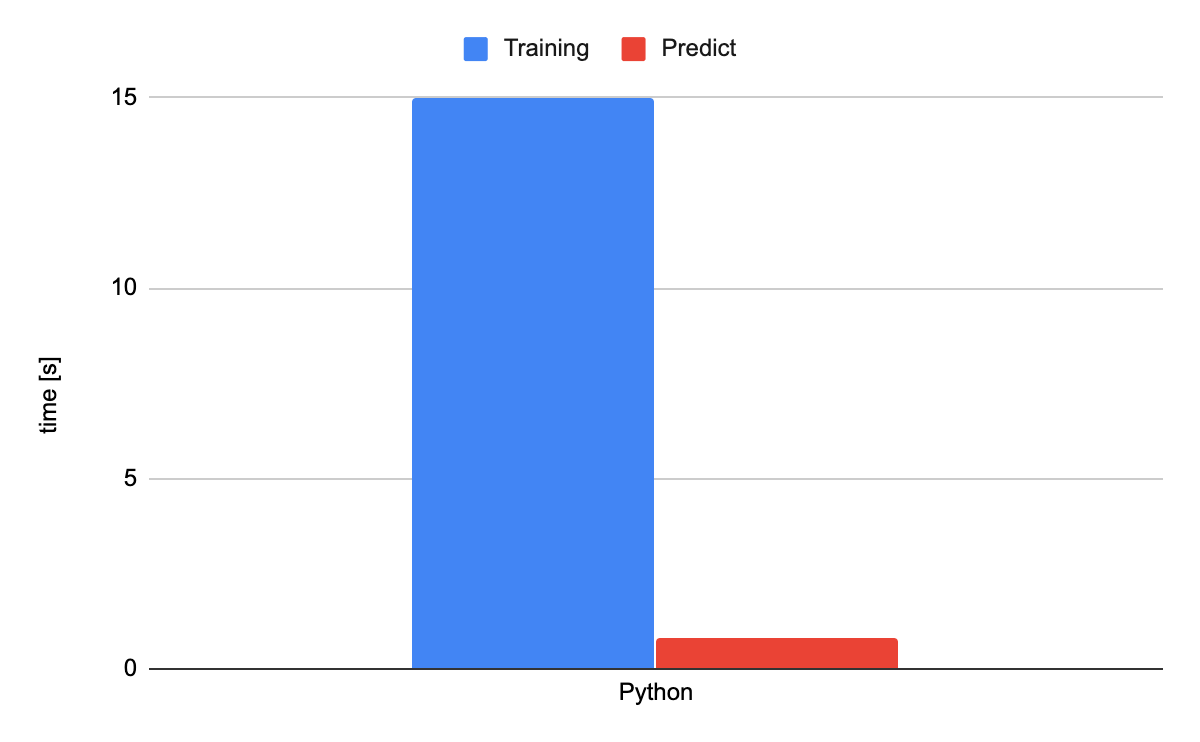Precise results: Python Training = 14.991 seconds — Python Prediction = 0.799 seconds

Same here as well: we only got Python results — JavaScript once again didn’t finish the process in the given timeframe.

### Neural network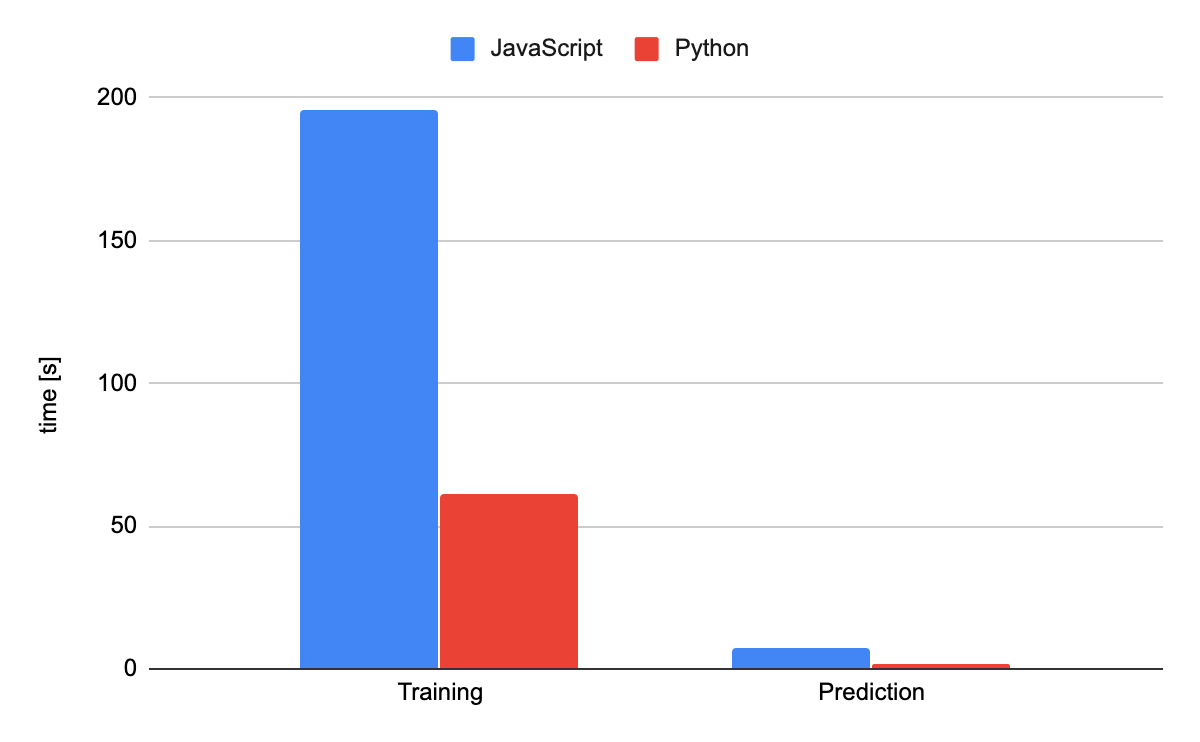Precise results — Training: JavaScript = 195.634 seconds — Python = 61.213 seconds
Precise results — Prediction: JavaScript = 7.366 seconds — Python = 2.030 seconds

## What Does It All Mean?

Sadly, I didn’t manage to test high-volume machine learning this time around. Still, the learnings from the tests I ran are stark. JavaScript couldn’t get close to Python’s tasks — across the board.

JavaScript’s computational performance is still much better than Python’s.

However, the maturity of the libraries — which often have underlying modules written in C — means that operations on large datasets can offer so much more than sheer computational power.

But there is still a place for JavaScript in machine learning. If you leverage ready-to-use models, you can cut the learning time and use resources just to make predictions. While if you already know how to code in JavaScript, it’s fine to use it as a basis to explore machine learning concepts.

Then, when performance becomes important, you can switch to Python.Krzysztof Miśtal

JavaScript Tech Lead focused on everything JS. He is also fascinated with cameras.### BERT SENTIMENT ANALYSIS ON VERTEX AI USING TFX

Learn how to apply BERT to sentiment analysis using TFX and Vertex AI pipelines.